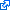# Adaptive Sparse Grids

Sparse grids come mainly in three flavors: Regular sparse grids, dimensionally adaptive sparse grids, and spatially adaptive sparse grids.

Regular sparse grids, as explained on the previous page (read up the notation if unclear), treat every dimension and every region of the domain $\clint{0, 1}^d$ as equally important. However, if the objective function $\objfun$ has different characteristics in the different dimensions or in specific regions of the domain, it might be good to use adaptivity to exploit these asymmetries of the objective function. A good adaptivity criterion generally leads to a smaller error with the same number of grid points (or fewer grid points are necessary to achieve the same error).

## Dimensional Adaptivity and Combination Technique

Regular sparse grids are constructed using a diagonal cut (diagonal line in 2D, diagonal hyperplane for general dimensionality) through the subspace scheme, which treats all dimensions equally. However, one dimension might be more important than another.

A simple example would be the bivariate objective function

$$\objfun(\*x) := \sin(20x_1) \cos(0.2 x_2),$$

which is a highly oscillating function along the first dimension and a very slowly oscillating function along the second dimension. Obviously, the first dimension is more important than the second, as few 1D grid points suffice to accurately resolve the slow oscillation along $x_2$.

This can be tackled with dimensionally adaptive sparse grids: Instead of cutting through the subspace scheme diagonally, we choose a different slope of the hyperplane such that the highest level in $x_1$ dimension is increased, while the highest level in $x_2$ dimension is decreased.

Dimensionally adaptive sparse grids, but also all sparse grids that are composed of whole hierarchical subspaces, can be treated with the sparse grid combination technique. The combination technique sees sparse grids as combinations of full grids instead of incremental grids.

For instance, the regular 2D sparse grid $\sgset{2}{2}$ of level two is the union of three full grids:

$$\sgset{2}{2} = \fgset{(2,0)} \cup \fgset{(1,1)} \cup \fgset{(0,2)}.$$

However, these full grids are not disjoint; therefore, some points are added twice. By counting, one can verify that the points added twice are exactly those points in

$$\fgset{(1,0)} \cup \fgset{(0,1)}.$$

When now shifting the perspective from grids to functions, the union of the three grids above turns into a sum, and the contributions from the two grids $\fgset{(1,0)}$ and $\fgset{(0,1)}$ have to be subtracted from that sum in order to incorporate every sparse grid point only once (so-called inclusion-exclusion principle).Combination technique to construct a regular sparse grid of level $n = 3$ in 2D (from , © by Julian Valentin, CC-BY-SA 4.0)

When reformulated with the combination technique, the function interpolant on the regular sparse grid $\sgset{n}{d}$ of level $n$ in $d$ dimensions becomes

$$\surfun(x) := \sum_{q=0}^{d-1} (-1)^q \binom{d-1}{q} \sum_{\normone{\*\ell} = n-q} \sum_{\*i=\*0}^{\*2^{\*\ell}} \coeff{\*\ell,\*i} \basis{\*\ell,\*i},$$

where the last sum is over $i_1 = 0, \dotsc, 2^{\ell_1},\; \dotsc,\; i_d = 0, \dotsc, 2^{\ell_d}$; it represents the full grid interpolant of level $\*\ell$. A similar formula exists for general dimensionally adaptive sparse grids.

The combination technique expresses the sparse grid interpolant in terms of full grid contributions instead of hierarchical contributions on the incremental grids. This means that existing algorithms that work on full grids (e.g., solvers of partial differential equations) may be reused to compute sparse grid solutions: The different and independent full grids can be processed in parallel, and at the end, the full grid solutions are combined according to the combination formula.

Dimensional adaptivity is useful, but sometimes it doesn’t suffice. Imagine a bivariate objective function like

$$\objfun(\*x) := \exp(-200 \cdot ((x_1-0.8)^2+(x_2-0.8)^2)).$$

Although this function is globally supported (it does not actually vanish), its values are almost zero in most regions of domain $\clint{0, 1}^2$ (except around $(0.8, 0.8)$, where it has relatively small peak). Regular or dimensionally adaptive sparse grids would have a lot of grid points in these regions, where the function is almost flat. As the corresponding hierarchical surpluses would almost vanish, this would be a waste of grid points. It would much more sense to place these points near $(0.8, 0.8)$, where the behavior of the function is more interesting.Construction of a spatially adaptive sparse grid in 2D (from , © by Julian Valentin, CC-BY-SA 4.0)

Spatial adaptivity makes this possible. Instead of whole incremental grids, spatial adaptive grids only choose a subset of the points of the incremental grids to avoid wasting points.

Often, spatially adaptive sparse grids are constructed iteratively. Starting with some initial coarse regular sparse grid, in each iteration a subset of the current grid points is chosen, and all children (neighboring grid points of the next higher level) of the grid points in the subset are inserted into the grid. In addition, there is usually some kind of consistency constraint on the resulting grid (e.g., the existence of all hierarchical ancestors of all grid points) such that sparse grid algorithms can still be applied.

There is a range of adaptivity criteria to choose the subset of grid points to be refined. The most popular criterion is the surplus-based criterion, which uses the absolute value $|\surplus{\*\ell,\*i}|$ of the hierarchical surpluses to estimate the second derivative of the objective function $\objfun$. A large absolute surplus $|\surplus{\*\ell,\*i}|$ usually corresponds to large absolute second derivatives of $\objfun$, which means that more grid points should be placed in the vicinity of $\vgp{\*\ell,\*i}$.

Literature: 

Learn more about different basis function types on the next page.J. Valentin, “B-Splines for Sparse Grids: Algorithms and Application to Higher-Dimensional Optimization,” PhD thesis, University of Stuttgart, 2019.D. Pflüger, Spatially Adaptive Sparse Grids for High-Dimensional Problems, Verlag Dr. Hut, 2010.